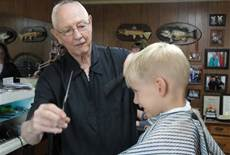# At the Barber's

Algebra Level 2You have two solutions of hydrogen peroxide: a $30$% solution and a $3$% solution and mix them to get a $12$% solution. If the ratio of the required amount of the two solutions is found to be in the form $\frac{a}{b}$, where $a$ and $b$ are coprime positive integers, then what is $a + b$?

×